# Ncert Class 6 Math Understanding Elementary Shapes Exercise 5.1

## Understanding Elementary Shapes Class 6 Chapter 5 Ex. 5.1

Exercise 5.1

Question;1:- What is the disadvantage in comparing line segments by mere observation?

Solution 1:- Chances of errors due to improper viewing are more.

Question 2. Why is it better to use a divider than a ruler, while measuring the length of a line segment?

Solution 2:- Measuring the length of a line segment using a ruler, we may have the following errors:
(i) Thickness of the ruler and
(ii) Angular viewing
These errors can be eradicated by using the divider.
For this reason, when determining the length of a line segment, a divider is preferable over a ruler.Question 3 :-Draw any line segment, say AB. Take any point C lying in between A and B. Measure the lengths of AB, BC and AC. Is AB = AC + CB?
[Note: If A, B, C are any three points on a line such that AC + CB = AB, then we can be sure that C lies between A and B]
Solution 3:-

Let us consider, A, B and C such that C lies between A and B.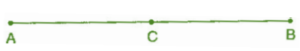Let’s say,

AB = 7 cm, AC = 3 cm, CB = 4 cm.
So, AC + CB = 3 cm + 4 cm = 7 cm.
But, AB = 7 cm.
So, AB = AC + CB.

Question 4 :- If A, B, C are three points on a line such that AB = 5 cm, BC = 3 cm and AC = 8 cm, which one of them lies between the other two?

Solution 4:- We have, AB = 5 cm; BC = 3 cm
AB + BC = 5 + 3 = 8 cm
But, AC = 8 cm
Hence, B lies between A and C.

Question 5 :- Verify, whether D is the mid point of AG.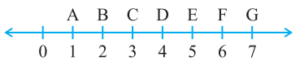Solution 5:- From the given figure, we have
AG = 7 cm – 1 cm = 6 cm
AD = 4 cm – 1 cm = 3 cm
and DG = 7 cm – 4 cm = 3 cm
So, AG = AD + DG.
Hence, D is the mid point of AG.(because, AD = DG = 3 units)

Question 6 :- If B is the mid point of AC and C is the mid point of BD , where A, B, C, D lie on a straight line, say why AB = CD?

Solution 6 :-

B is the mid point of AC.
So,  AB = BC                       …(i)
C is the mid-point of BD.
BC = CD                             …(ii)
From Eq.(i) and (ii), We have
AB =CD.

Question 7. Draw five triangles and measure their sides. Check in each case, if the sum of the lengths of any two sides is always less than the third side.
Solution 7 :-

Case I. In ∆ABC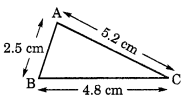Let AB = 2.5 cm, BC = 4.8 cm and AC = 5.2 cm
AB + BC = 2.5 cm + 4.8 cm
= 7.3 cm
Since, 7.3 > 5.2
So, AB + BC > AC
Hence, sum of any two sides of a triangle is greater than the third side.

Case II. In ∆PQR,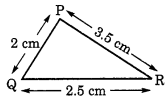Let PQ = 2 cm, QR = 2.5 cm and PR = 3.5 cm
PQ + QR = 2 cm + 2.5 cm = 4.5 cm
Since, 4.5 > 3.5
So, PQ + QR > PR
Hence, sum of any two sides of a triangle is greater than the third side.

Case III. In ∆XYZ,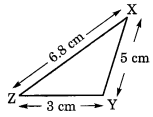Let XY = 5 cm, YZ = 3 cm and ZX = 6.8 cm
XY + YZ = 5 cm + 3 cm = 8 cm
Since, 8 > 6.8
So, XY + YZ > ZX
Therefore, the sum of any two triangle sides is greater than the sum of the third side.

Case IV. In ∆MNS,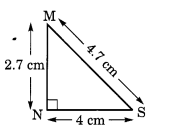Let MN = 2.7 cm
NS = 4 cm
MS = 4.7 cm
and MN + NS = 2.7 cm + 4 cm = 6.7 cm
Since, 6.7 >4.7
So, MN + NS > MS
Therefore, the sum of any two triangle sides is greater than the sum of the third side.

Case V. In ∆KLM,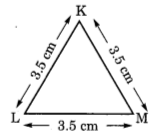Let KL = 3.5 cm, LM = 3.5 cm and KM = 3.5 cm
and KL + LM = 3.5 cm + 3.5 cm = 7 cm
7 cm > 3.5 cm
So, KL + LM > KM
Therefore, the sum of any two triangle sides is greater than the sum of the third side.

Therefore, we draw the conclusion that the total of any two triangle sides is always greater than the third side.